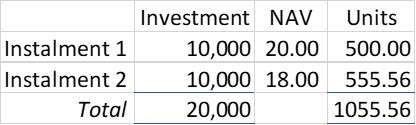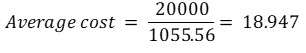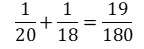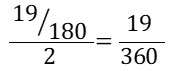# Why SIPs work better: The science behind cost averaging

We all have our ‘eureka’ moment and mine came while buying mangoes. Yes, buying mangoes helped me explain why average costs come down more than you expect it to, when investing through a SIP.

One of the key benefits of SIP is said to be cost averaging but it is not easy for investors to understand how SIP averaging is different from what you would do in your daily life why buying more of a same thing.

Let us say you invested ₹10,000 in a fund when the NAV was ₹20. By the next month, the NAV of the fund fell to ₹18 and you invested another ₹10,000 at this point. What would be the average cost of your units? Intuition would tell you that it would be ₹19. But look at this calculation:What happened there? The average cost of your units is ₹18.947, and not ₹19! How is that?

Let us just keep aside the above calculation for a minute and take this completely unrelated example.

Just like I did, let us say you too went to the market to buy 2 KG of mangoes, expecting them to be selling for ₹80 for a KG. But to your utter dismay, you found the price to be ₹120 per KG. Disappointed, you decided to buy only 1 KG. But as you were walking back, you found another cart selling the same mangoes for ₹60 a KG. You were elated and bought another KG of mangoes.

What was the average cost of the mangoes?

This time it is really simple. The average cost per KG is (120 + 60) / 2 = ₹90. Just as your intuition would tell you.
So what is the difference between this and the SIP?

When you went out to buy the mangoes, you bought the same quantity of mangoes at different rates. Hence you paid a smaller sum where the rate was lower. But when you were investing in SIP, you invested the same amount, regardless of the lower NAV. This means you bought a higher number of units at the lower cost.

To take the above example once again, suppose instead of buying 1 KG at the second cart, you again bought mangoes for ₹120. You would end up with 3 KG of mangoes and your average per KG cost would have been ₹80 (and not ₹90), and you would happily walk back home, having bought the mangoes for the expected price.

This is exactly what is happening in the SIPs. You are not buying the same number of units for a lower cost when the market is falling. You are buying a higher number of units for a lower cost. Thus your average cost comes down more than you would expect it to.

Mathematically speaking, the average cost of your units can be arrived at by taking the harmonic mean of all the NAVs at which you invested. Harmonic mean is essentially the reciprocal of the average of reciprocals of all your numbers. You can read more here: Harmonic Mean – Wikipedia.

So to calculate your average cost from the first example, you would sum the reciprocals of the NAVs, i.e.:You would then divide it by the number of observations, i.e.:You would then take the reciprocal of this, i.e.:And this gives you the average cost of your units.

Harmonic mean might not be as intuitive as regular average, but it answers your questions on average rates. And the above example shows us that when you invest through SIP, cost averaging works better than you would normally expect it to. And this, therefore, is one more reason why we recommend SIPs to our investors.

This article first appeared in the online edition of Financial Express.